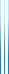# PublicationsManuscripts
If $(x,y)=1$ then $(x+y,xy)=1$. The first part shows how to prove this using standard arguments and using the characterization of GCD as the minimal positive value obtained as an integer combination of the operands. The second part generalizes the argument to a lemma involving $n$ variables.